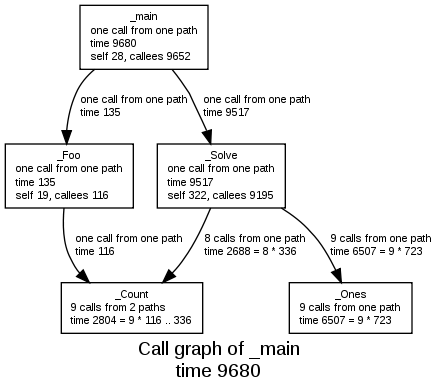### Call graph from Bound-T analysis

The call graph shows all the subprograms included in an analysis and all the call relationships between these subprograms in the worst-case execution scenario found by the analysis.Each box in the drawing represents a subprogram and is labelled with:

• the name of the subprogram,
• the number of calls of the subprogram (in the worst-case execution),
• the number of call paths that lead to the subprogram (in the worst-case execution), and
• the WCET bounds of these calls.

The WCET bounds are shown both in total (sum of all calls) and per call, and also broken down into the WCET bound for the code in this subprogram itself ("self") and the WCET bound for the callee subprograms ("callees").

Each arrow represents a call relationship (from caller to callee) and is labelled with:

• the number of calls,
• the number of call paths and
• the WCET bounds for these calls.

There are two forms of this drawing: the default form, which is shown above, and the "bounds graph" form which is chosen with the command-line option "-draw bounds". In the bounds-graph form, if a subprogram has context-dependent WCET bounds (that is, different WCET bounds for different call paths) each context is shown as its own box. The subprogram appears in the drawing in as many boxes as it has context-dependent bounds. Look here for the bounds-graph of this example.